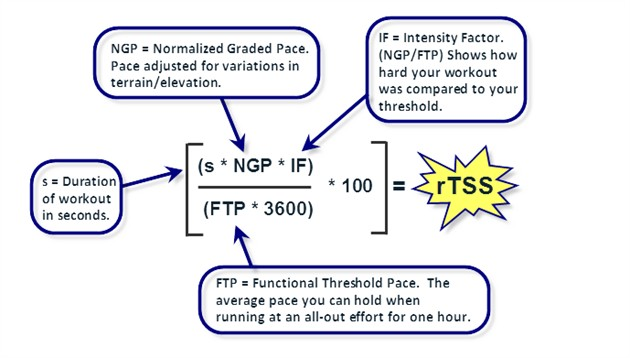トライアスロン

## How is rTSS calculation of Trainingpeaks carried out: About correction of rTSS

How to calculate rTSS of Trainingpeaks is described like this.

Running Training Stress Score (rTSS*) Explained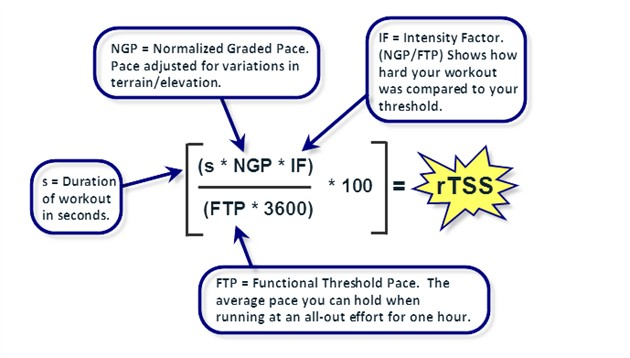When this formula is transformed, rTSS = 100 x Duration[hrs] x IF[-]^2, IF = NGP/FTp

NGP stands for Normalized Graded Pace, which is a value obtained by correcting the average pace with a slope.

NGP will be faster than Average Pace (AP) if the ratio of climbing on the road is large, NGP will be slower than AP if the ratio of descent is large.

FTp represents the Functional Threshold pace, which is the same concept as Bike’s FTP, namely the threshold pace.

Let’s calculate using a concrete example.

Consider the case where FTp people（4:30min/km） ran in NGP（５:00min/km）.

Since IF is the ratio of speed, take the reciprocal of the above “time per km”

IF = (1/5: 00) / (1/4: 30) = (1/300) / (1/270) = 270/300 = 0.9

By the way, if I ran 30 minutes in this case, how many rTSS would you have?

rTSS = 100 x 0.5 x 0.9 ^ 2 = 40.5, is not it?

No, it is different.

If Trainigpeaks makes the same calculation, it should calculate a larger number than this rTSS. But, the calculation formula is not wrong …

That’s right, Probably this estimation formula is incorrect </ strong>.

P.289 of Power Training Bible leaves the following description.

100TSS by Bike is the same as 100rTSS in Run.
Since Run is a movement that bounces the ground with legs, the load on the musculoskeletal system is more than Bike (gravity acting according to body weight when the foot lands) is applied.

If you run at Ftp (FT pace) for 1 hour in a run, it will take higher training stress than a 1 hour ride with Bike, so it takes longer to recover.
So it is equivalent to one hour run by FTP in Bike about 45 minutes running at FTp in a Run. (Partial description change)

With reference to this description, I tried 60/45 = 1.333, that is, I increased the rTSS I got earlier by 33%, but in that case the rTSS would be too large.

Probably “about 45 minutes” is not 45 minutes.

So I tried to derive the formula for the correction term C from my actual training value. The correction term C is as follows.

rTSS-C = 100 x Duration[hrs] x IF[-]^2 x C, IF = NGP/FTp

Because it becomes an empirical formula, the scope of application is clarified.

＜Premises＞
FTp・・・4:42min/km
Duration・・・from 20 minutes to 5 hours 51 minutes
Number of data・・・１０点
NGP・・・Calculation results of Traingpeaks

I am using Garmin 735xtj to measure run data, but it seems that Elevation data is not accurate.
There is a problem of estimating the height difference of the topography bigger than it actually is.
So, when calculating NGP, if NGP is the same as actual climbing and descent ratio, NGP will be the same, but there is a possibility that it is not necessarily so.

Here we treat any NGP as including similar errors.

To put it briefly, the absolute amount of NGP itself is doubtful because the data on the height difference of the terrain is doubtful, but the data is ignored since the suspiciousness is the same between the data.

Take the ratio of rTSS and rTSS – C led by Trainig Peaks to obtain C = rTSS – C / rTSS.

From the quotation above, I thought that “If the running time is long, the musculoskeletal will be fatigued by gravity,” we approximated time with x and C value in y and approximated by a logarithmic function.

Then …

Apparently, an approximate line has been made with quite good precision.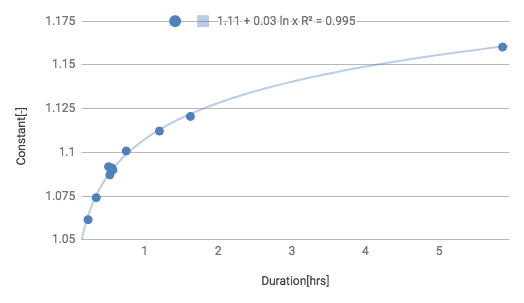y = 0.03ln(x) + 1.1074

By applying this expression to rTSS – C = 100 x Duration [hrs] x IF [-] ^ 2 x C and verifying it matches the calculated value of Training Peaks with high accuracy.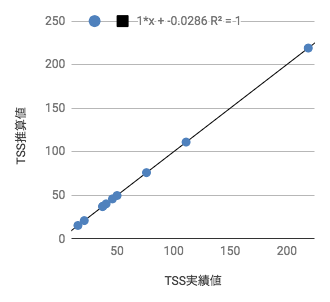When qualitatively grasping this correction term C,

· In the above “quotation”, about 45 minutes of running FTp is written as equivalent to bike FTP 1 hour, but if it is my FTp premise it will be 50-56 minutes equivalent.

· When comparing run training of 20 minutes and 60 minutes, the C value differs by 5%, but it becomes 1% difference between 1 hour and 2 hours

· As the run time becomes longer, accumulation of muscle fatigue increases and the rTSS increases, but its increase becomes moderate

Incidentally, even though the intensity is high, I thought that musculoskeletal fatigue might be promoted, and I also took a correlation between IF and C.
However, there was no correlation. (The correlation coefficient is not given, but it will not be repeatable at the approximate expression.)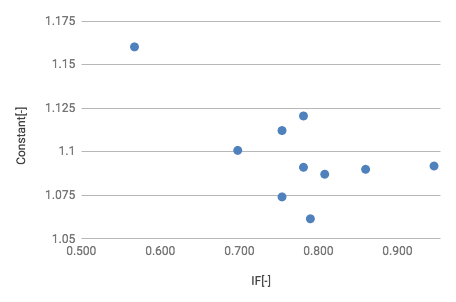### Conclusion

rTSS can be calculated as follows.

rTSS = 100 x Duration[hrs] x IF[-]^2 x （0.03ln(Duration) + 1.1074）

Scope of application
0.333<Duration<6
FTP = nearly 4:42min/km

If you are not using Trainingpeaks’ software, please incorporate this correction to see if you can trace the results of calculation so far and use it if there is no problem.

Please tell me who is faster than my FTp (data is better away) and if you have data.
I will verify.

See you soon！

### 関連記事

1.トライアスロン

#### 海外ロングレース大会に向けた準備品のまとめ

ロングレースに必要なアイテムを一覧表にまとめました。一部はもち…

2.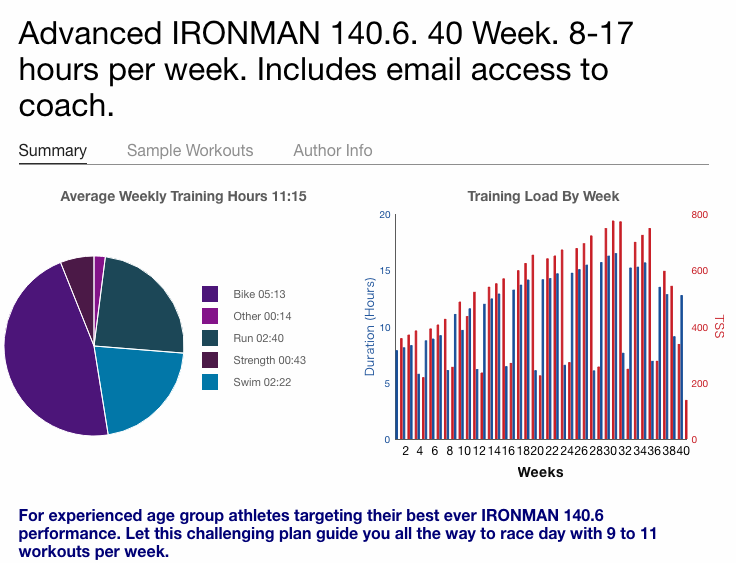トライアスロン

#### Annual training plan for Ironman race created! Thi…

The date of the Goto Nagasaki Inter…

3.トライアスロン

#### 疲労が蓄積しているときにトレーニングをすべきか休むべきか。

みなさん、こんばんは！6月のレースまであと9weeks、技術士トラ…

4.トライアスロン

#### トライアスロンレースのダメージに関する考察

あるディスタンスレースにおけるトライアスロンのダメージは、選手のレベル…

5.トライアスロン

#### ランのインターバル練習で理想的なランニングフォームを思い出せた話

ランのトレーニングで気づきがあったので書き留めておきます。インター…

6.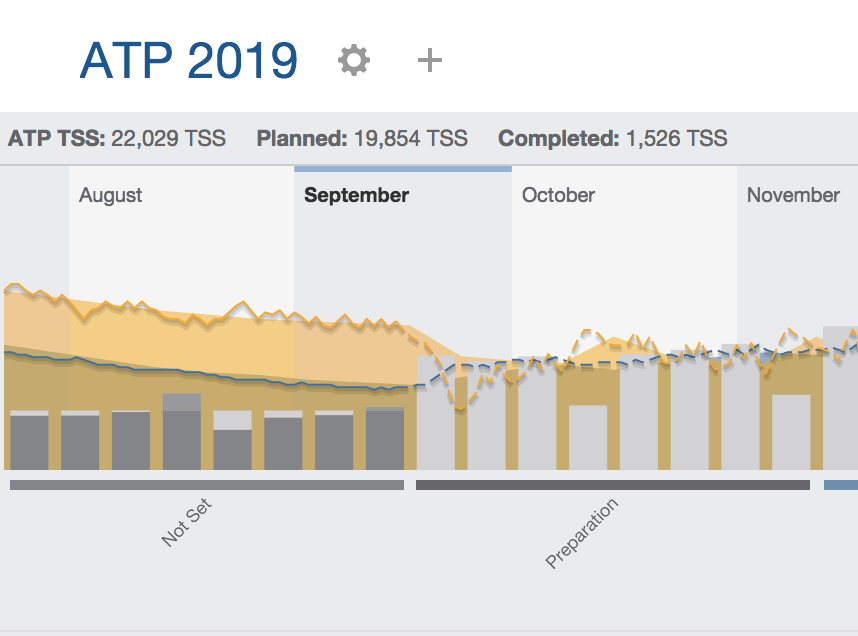トライアスロン

#### アイアンレースに向けたボディメイクにトライアスロントレーニングを組み込んでいきます！

ボーナス期間終了！トライアスロンのトレーニングを開始します！ということ…